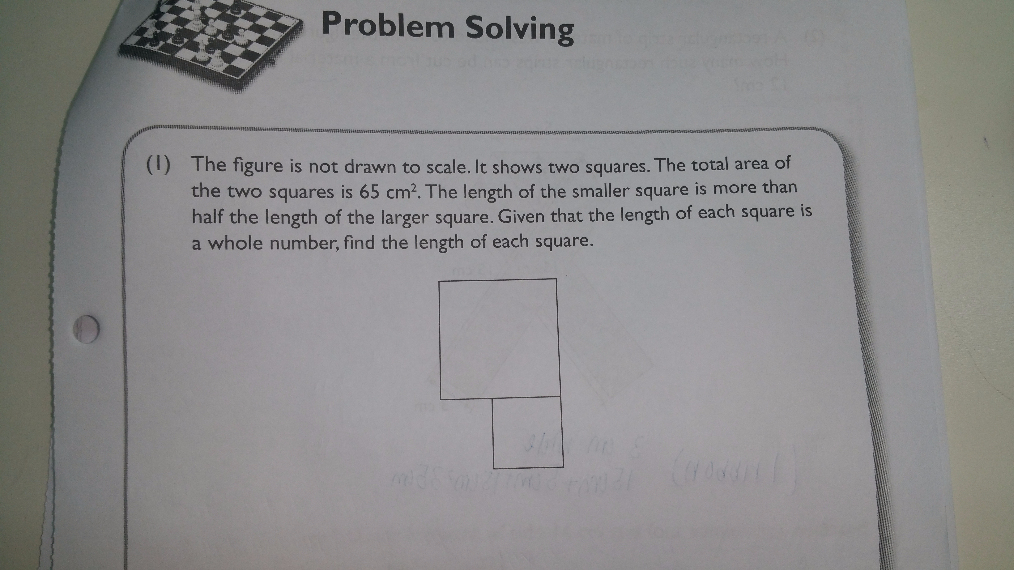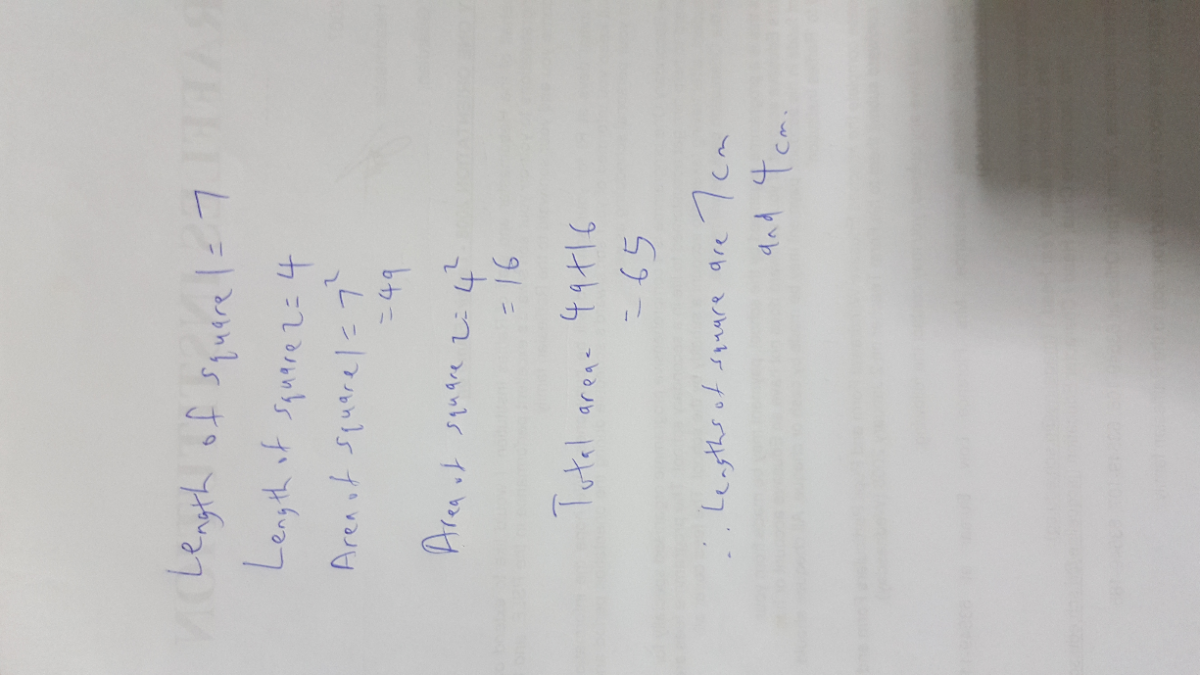# QuestionHi I would appreciate it a lot if I could get help with this question. thank you! 🙂

List of square numbers : 1, 4, 9, 16, 25, 36, 49, …
65 = 16 + 49
16 = 4 x 4
49 = 7 x 7

Ans : 4 cm and 7 cm.

0 Replies 0 Likes

I would just use smart guess and check.

What is smart guess and check?

Instead of just choosing 2 random numbers and check if their squares add up to 65, analyse the question first to determine possible combinations.

First, √65 = 12 + 82

But since it was stated that the length of the smaller square is more than half of the length of the bigger square, this is not an acceptable answer. How it helped was that we now know the length of the bigger square must be shorter than 8cm.

Since 8 ÷ 2 = 4

We now know that the length of the smaller square is either 2cm, 3cm, or 4cm.

If 2cm, 22 = 4, 65 – 4 = 61, √61 is not whole number.

If 3cm, 32 = 9, 65 – 9 = 56, √56 is not whole number.

So length of smaller square must be 4cm.

42 = 16

65 – 16 = 49

√49 = 7

The lengths of the squares are 4cm & 7cm

0 Replies 0 Likes0 Replies 0 Likes